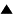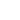# Infinity

[Node to be completed]

In mathematics and philosopy we find two concepts of infinity: potential infinity, which is the infinity of a process which never stops, and actual infinity which is supposed to be static and completed, so that it can be thought of as an object.

The cybernetic philosopy readily embraces the concept of potential infinity; in fact, it is hard to see how we can avoid it. We say that a process ends when it reaches a certain stage. In a particular case, we can define the end stage so that it never takes place. As every general notion, this is an abstraction: we have abstracted from the practical impossibilty to run any real process infinitely, and in fact, for very long. In this abstraction, no matter how long we have run the process, we always can do, or observe, the next step. This is why this infinity is called potential. At every specific stage the process involves no more than quite a finite reality; it is infinite only potentially.

For actual infinity we have no place in our system of concepts. On the intuitive level, we cannot imagine anything that would qualify as actual infinity, because neither we, nor our evolutionary predecessors never had anything like that in experience. When we try to imagine something infinite, e.g., infinite space, we actually imagine a process of moving form point to point without any end in sight. This is potential, not actual, infinity.

On a more formal level we can demonstrate the incompatibility of the concept of actual infinity with our cybernetic understanding of meaning. Indeed, suppose that some abstraction r represent the concept of an "infinite" object, and we use it while constructing a model. According to our semantics, there must exist an abstraction (representation) function F which recognizes whether a given state of the world belongs to this concept, an if so, results in r. Moreover, function F must, by definition, always require a finite time for coming up with a definite result. If the "infinite" object can be always recognized as such in a finite number of steps, it is not actually infinite, because it can be adequately replaced by a finite object. If, by the intuitive meaning of the "infinite" object r its recognition may require infinite time, then abstraction function will have to work, at least in some cases, infinitely, but then it is not a valid abstraction function. Thus we cannot use the concept of actual infinity at all.

As an example, consider the process of counting. We can imagine it going on infinitely if we do not count real sheep or apples, but simply produce concecutive numbers. Let us represent numbers (whole and positive) by strings of the symbol '1'; then the process is:

1, 11, 111, 1111, ... etc. infinitely.

When we say that this process is infinite, we mean that whatever is the current number, we can add one more '1' to it. Thus we deal with potential infinity.

To convert it into an actual infinity, we must imagine an object that includes in itself all whole numbers. We call it the set of all positive whole numbers. Suppose that such a thing exists. How would it be possible for an abstraction function F to distinguish it from other objects, e.g. from the set of all whole numbers with the exception of the number 10^{50}? Intuitively, F must examine the infinite number of the elements of the set. Since this is impossible to achieve in any finite time, the needed function F does not exist.

What we can do, however, is to create an objectification of the process which generates all whole numbers. A machine which initiates this process (and, of course, never stops) is such an objectification. This machine is a finite object. It can be made of metal, with an electric motor as the agent causing the process of work. Or we can describe it in some language addressing a human agent, but requirung only simple "mechanical" actions uniquely defined at each stage of the process. Such descriptions are known as {\algorithms}. If we use the English language for writing algorithms, the machine, to be referred to as N, could be as follows:

At the initial stage of the process produce the number '1'. At each next stage take the number produced at the preceding stage and produce the number obtained by adding '1' to it."

Now we can say that the set of whole numbers is N. We have no objections against sets defined in this way. Their meaning is crystal clear. Their infinity is still potential.

The problem with contemporary mathematics is that it operates with sets that cannot be represented by finite mechanical generators. They are uncountable.

The question of the nature and meaning of these sets is, in the eyes of contemporqry mathemticians and philosophers, wide open. Yet their usefulnes is abundantly demonstrated, and everybody believs that their use will never lead to contradiction. Thus it is important for us to interpret uncountable sets -- and the whole set theory -- in terms of our cybernetic, constructive philosophy. If we were unable to do this, it would undermine our claim that the basic principles on which we build our philosophy are universally applicable and sufficient.

Fortunately, we can interpret set theory, as well as classical and intuitionist logic, in our terms (see Foundations of logic and mathematics). Our interpretation assigns quite definite meanings, in our sense of the word, to the basic concepts of logic and set theory, and does it without any recourse to the concept of actual infinity.

 HomeMetasystem Transition TheoryMetaphysicsBasic Concepts of Science Up Prev.Next Down